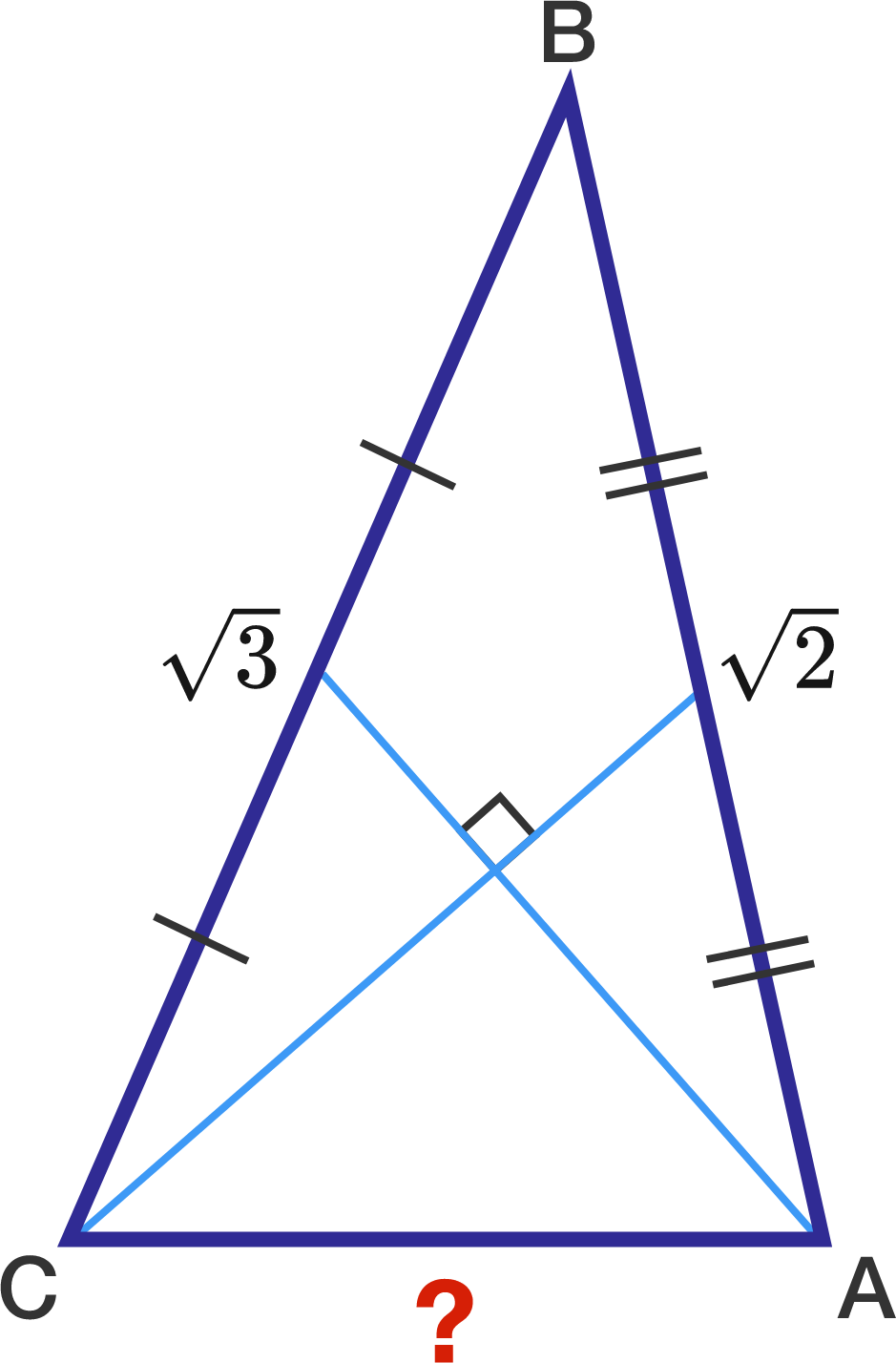# A geometry problem by Kshetrapal Dashottar

Geometry Level 3The lengths of two sides of a triangle are $\sqrt{3}$ and $\sqrt{2}$.
The medians to these sides are perpendicular to each other.

Find the length of the third side.

×